Ex 8.2

Chapter 8 Class 9 Quadrilaterals
Serial order wise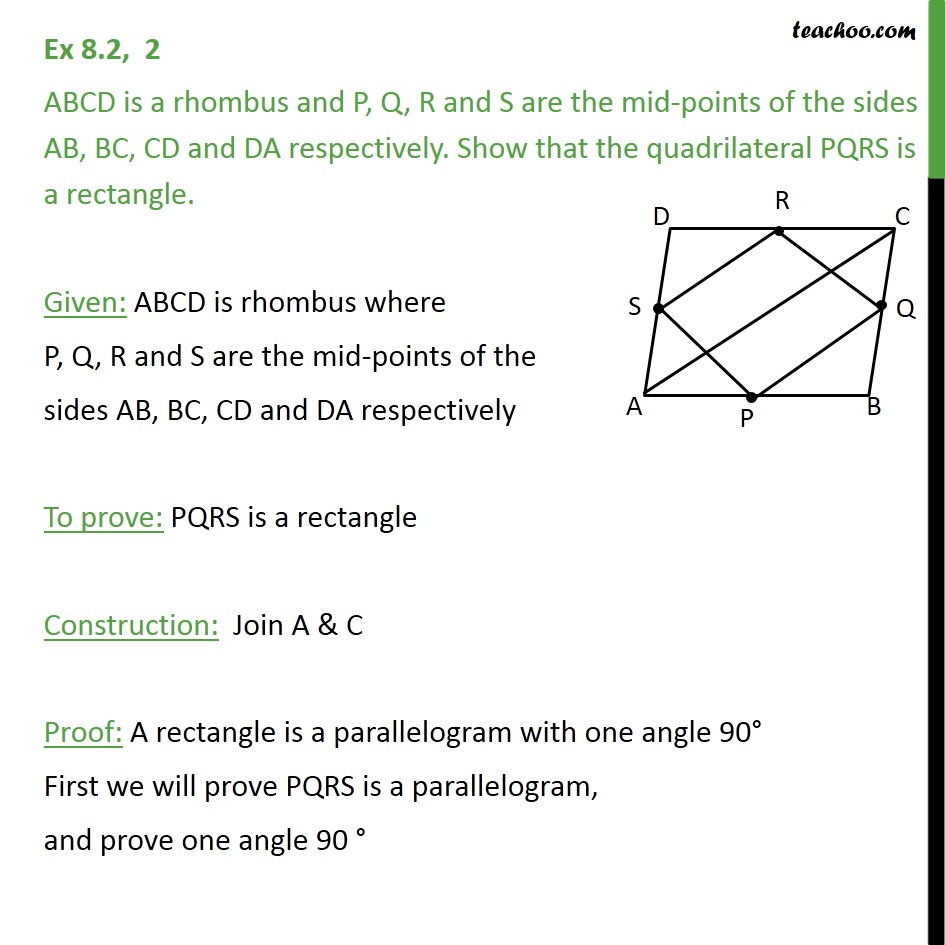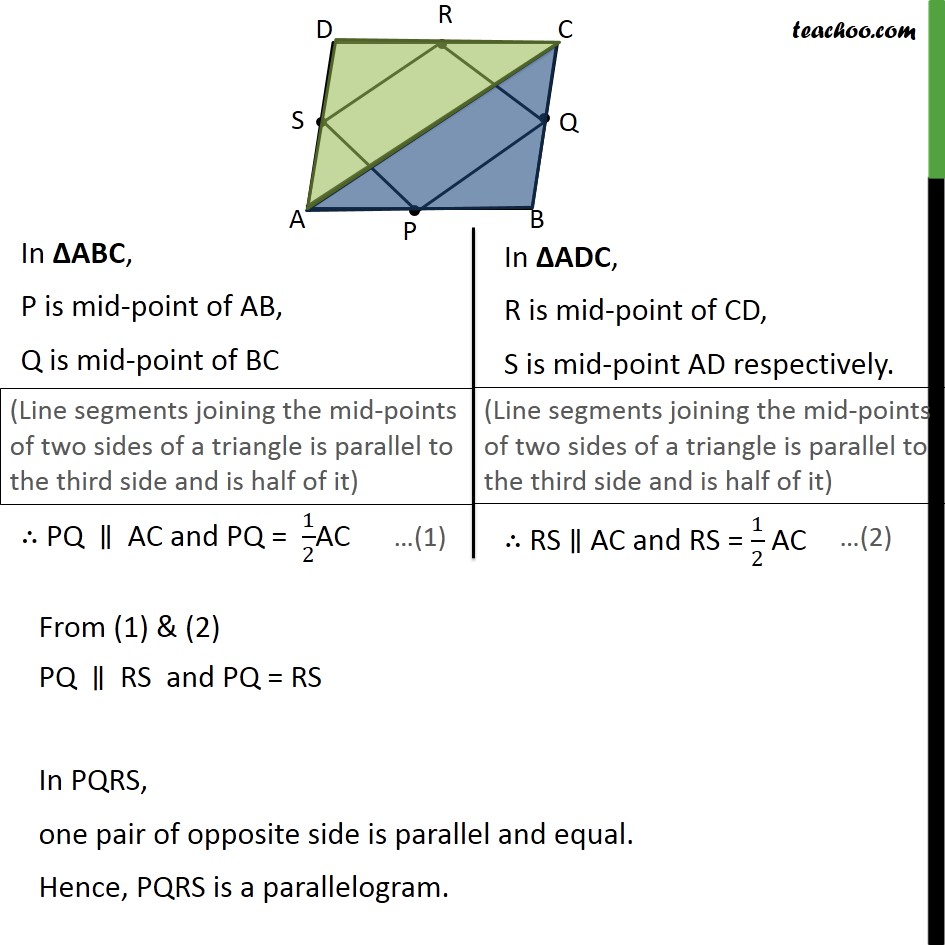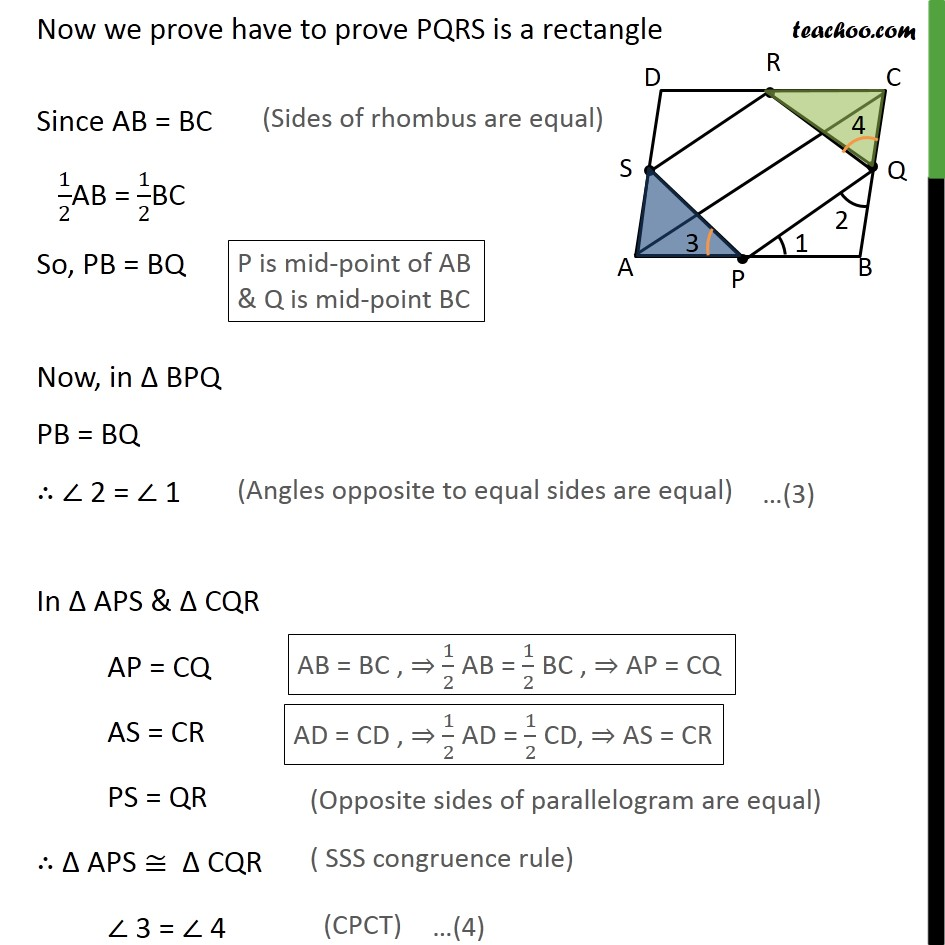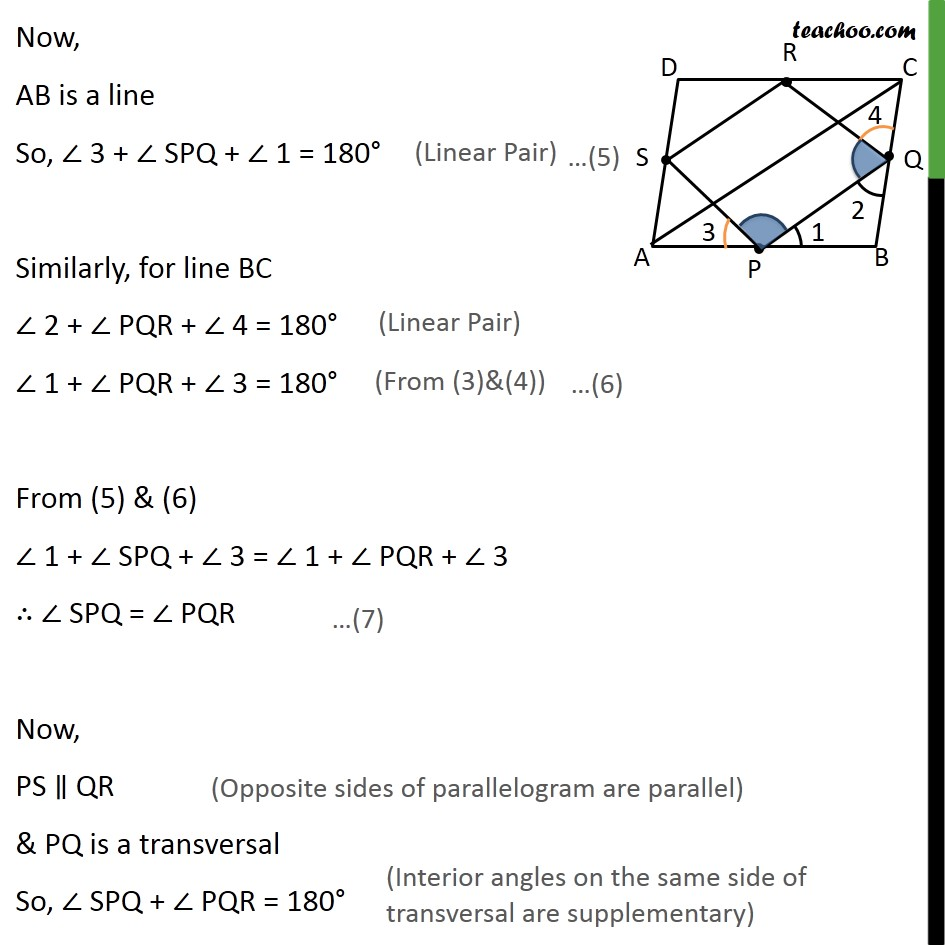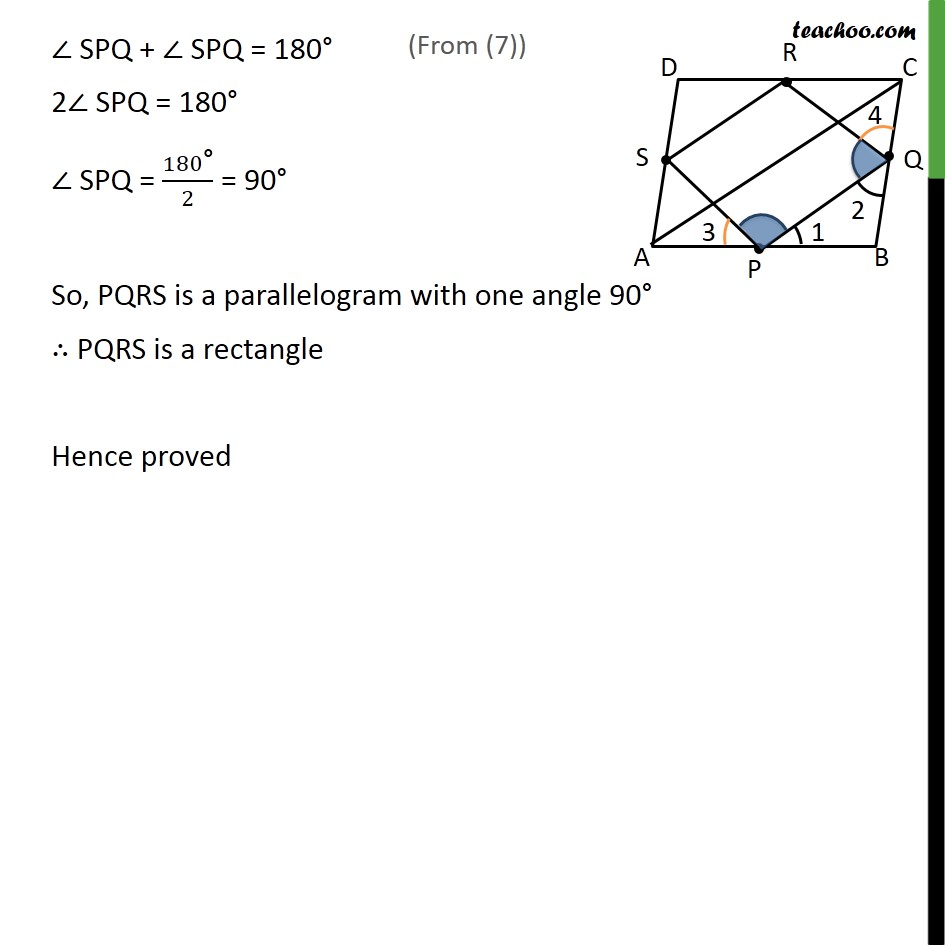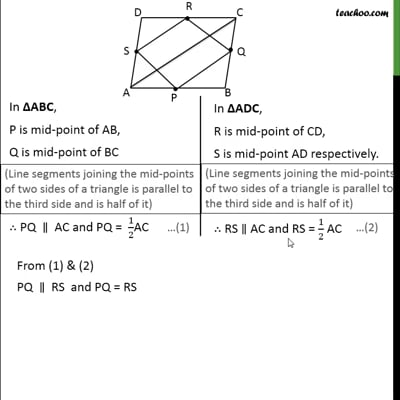This video is only available for Teachoo black users

Solve all your doubts with Teachoo Black (new monthly pack available now!)

### Transcript

Ex 8.2, 2 ABCD is a rhombus and P, Q, R and S are the mid-points of the sides AB, BC, CD and DA respectively. Show that the quadrilateral PQRS is a rectangle. Given: ABCD is rhombus where P, Q, R and S are the mid-points of the sides AB, BC, CD and DA respectively To prove: PQRS is a rectangle Construction: Join A & C Proof: A rectangle is a parallelogram with one angle 90° First we will prove PQRS is a parallelogram, and prove one angle 90 ° From (1) & (2) PQ ∥ RS and PQ = RS In PQRS, one pair of opposite side is parallel and equal. Hence, PQRS is a parallelogram. Now we prove have to prove PQRS is a rectangle Since AB = BC 1/2AB = 1/2BC So, PB = BQ Now, in Δ BPQ PB = BQ ∴ ∠ 2 = ∠ 1 In ∆ APS & ∆ CQR AP = CQ AS = CR PS = QR ∴ ∆ APS ≅ ∆ CQR ∠ 3 = ∠ 4 Now, AB is a line So, ∠ 3 + ∠ SPQ + ∠ 1 = 180° Similarly, for line BC ∠ 2 + ∠ PQR + ∠ 4 = 180° ∠ 1 + ∠ PQR + ∠ 3 = 180° From (5) & (6) ∠ 1 + ∠ SPQ + ∠ 3 = ∠ 1 + ∠ PQR + ∠ 3 ∴ ∠ SPQ = ∠ PQR Now, PS ∥ QR & PQ is a transversal So, ∠ SPQ + ∠ PQR = 180° ∠ SPQ + ∠ SPQ = 180° 2∠ SPQ = 180° ∠ SPQ = 180"°" /2 = 90° So, PQRS is a parallelogram with one angle 90° ∴ PQRS is a rectangle Hence proved